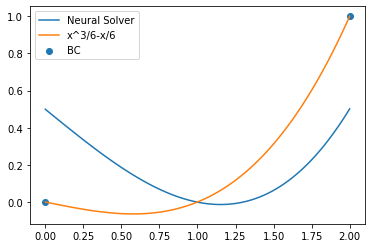# Basic Custom PDE - problem with boundary conditions

As a beginner in using the Modulus framework, I tried to follow the initial code given in the following link:

https://docs.nvidia.com/deeplearning/modulus/user_guide/notebook/notebook.html

In order to learn, I tried to change the existing code in order to solve the equation of the form u__x__x = x with boundary conditions (BC):
u(0) = 0 and u(2)=1, where x in [0, 2]. Considering that the analytical solution of this equation is u = x*(x^2-1)/6, unfortunately, I did not get even an approximate result using Modulus.

In order to set the appropriate BC, I made the following change:

from modulus.domain.constraint import PointwiseBoundaryConstraint, PointwiseInteriorConstraint

# bcs

bc = PointwiseBoundaryConstraint(
nodes=nodes,
geometry=geo,
outvar={“in”: (0, 1)},
batch_size=2,
)

Considering that I don’t have a good results, can the BC conditions: u(x=0) = 0 and u(x=2) = 1 be set with outvar={“u”: (0, 1)}?

Result is shown below:For two different boundary conditions I would suggest you create two different constraints. I.e. one on the left and right. You can use the criteria parameter of the boundary constraint to specify if its left or right. See the LDC script as an example. E.g.

``````left_bc = PointwiseBoundaryConstraint(
nodes=nodes,
geometry=geo,
outvar={“in”: 0},
criteria=Eq(x, 0),
batch_size=2,
)